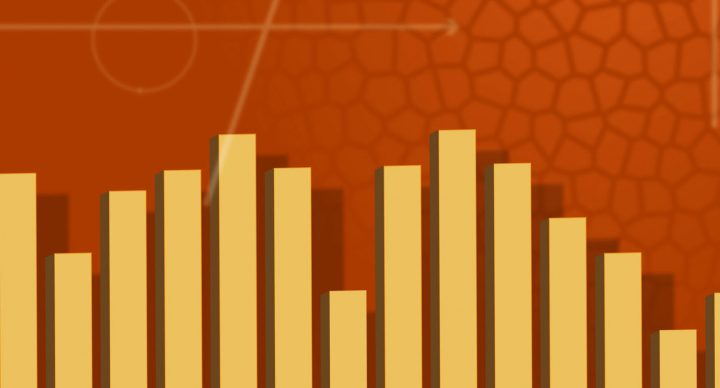# Basic Math Concepts for Middle School### Course Overview

This remedial math course is recommended for middle school students with very weak math skills.  It presents the lesson material on a 3rd grade level.  This course covers math fact memorization and basic concepts. Basic Math Concepts for Middle School is taught by Acellus Instructor Latricia Harper.This course was developed by the International Academy of Science. Learn More

### Scope and Sequence

Unit 1 In this unit students gain understanding of addition, subtraction, and fact families. They also learn about sums and differences, story problems, and working with addends. Unit 2 In this unit students explore digits. They begin to understand place value up to three digits, as well as expanded form and word form of numbers. They also explore number order using number lines and counting order. They investigate odd and even numbers, and learn to compare larger and smaller numbers. They study counting by numbers other than one. Unit 3 In this unit students expand their understanding of place value up to four digits and the thousands period. They explore expanded and word forms, as well as comparing and ordering numbers. Unit 4 Using their knowledge of three- and four-digit numbers, in this unit students gain a thorough understanding of rounding, including rounding to tens, hundreds, and a given place value. They also explore counting by numbers beyond the number one, and using benchmarks. Unit 5 In this unit students investigate mental sums and estimation, including using various place values, using compatible numbers, and doing mental sums and story problems. They also study addition's properties and adding with parentheses or compatible numbers. Unit 6 In this unit students consider aspects of addition such as adding with two- to four-digits, and using regrouping. They also explore deciding on a method and doing story problems. Unit 7 Once again exploring mental math and estimation, in this unit students focus on differences, including tens, hundreds, thousands, and story problems. They consider compatible numbers, over-and under-estimating, and deciding between estimation and exact calculation. Unit 8 In this unit students review subtraction, studying two- through four-digit subtraction, regrouping, and using addition to check subtraction. They explore subtracting with different place values, and finding differences across zeros. They also practice story problems with both addition and subtraction. Unit 9 In this unit students focus on handling money. They learn to compare money, to count money, and to add and subtract money. They do money story problems, learn to make change, and celebrate the number 100. Unit 10 In an introduction to statistics, students use this unit to study ten different kinds of graphs. They learn about line and circle graphs, line plots, bar and double-bar graphs, Venn diagrams, pictographs, tally and frequency tables, and using a coordinate grid. Unit 11 With lessons on multiplying by the numbers 0-11, the properties of multiplication, finding missing factors, and calculator multiplication, in this unit students gain a solid foundation in multiplication. They also discuss solving problems with tables and practice story problems. Unit 12 In this unit students develop their understanding of division. They learn to divide by the numbers 0-12, fact families, multiples of two, three, and four, and division story problems. They explore operations, including order and selection, using a calculator for division, equations, and expressions. Unit 13 In this unit students study one- and two-dimensional shapes, including lines, polygons, angles, triangles, circles, quadrilaterals, and congruent figures. They discuss symmetry. They explore three-dimensional shapes and how they relate to objects. Unit 14 In this unit students investigate measuring with customary and metric units, including capacity, length, mass, and weight. Following this unit students are presented with the Mid-term Review and Exam. Unit 15 In this unit students delve into understanding fractions, beginning with modeling. They learn about numerators and denominators, simplest form, comparing fractions, whole numbers as fractions, number lines, and fractional equivalents. Unit 16 In this unit students expand their understanding of fractions to include mixed numbers. They practice converting between fractions and mixed numbers, comparing mixed numbers, and adding, subtracting, and multiplying mixed numbers and fractions. They explore how mixed numbers are used on a number line, and practice doing mixed number story problems. Unit 17 In this unit students study decimals. They learn to relate decimals to mixed numbers and fractions, to add and subtract decimals, and to use decimals on a number line. They also study thousandths and practice story problems. Unit 18 In this unit students deepen their understanding of multiplication. They explore regrouping, mental products, two-and three-digit multiplication story problems, selecting a multiplication method, and estimating products. Unit 19 Continuing their exploration of multiplication, in this unit students learn to multiply numbers with up to four digits by numbers with up to two digits. They study how zeros and tens work in multiplication, learn multiples of eight, and work out story problems. Unit 20 In this unit students expand their understanding of division. They focus on dividing two digits, both with regrouping and through story problems. They explore mental quotients, sharing evenly, and using models. They learn to estimate quotients and to use multiplication to check division. Unit 21 Continuing to study division, in this unit students explore how to divide up to three-digit numbers by one-digit numbers and how remainders work. They look at where to place the first digit, special topics in division, and how zeros work in division. Unit 22 In this unit students further study division. They investigate dividing up to four-digit numbers with two-digit numbers, practice real-world story problems, explore how to check division using multiplication, choosing a division method, and adjusting quotients. Unit 23 In this unit students learn about area, volume models, and perimeter. They also study volume measurements and metric capacity units. Unit 24 In this unit students investigate negative numbers, number patterns, and comparing with negative numbers. They also study factors, divisibility rules, prime numbers, multiples, and composite numbers. Following this unit students are presented with the Final Review and Exam.